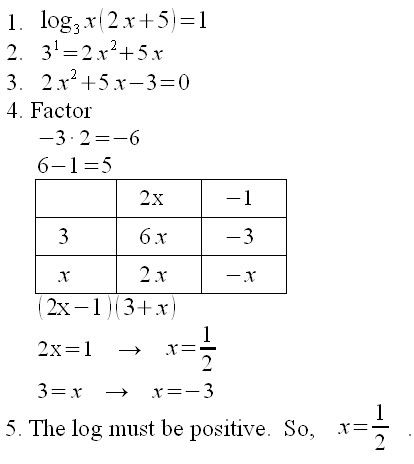# Calculus Test 1

1. Specify the domain of the function y = |x – 1|.

2. Which of the following is a true statement about the graph of the equation$y = x^4 +1$?
3. 1. It is symmetric about the x-axis.
2. It is symmetric about the y-axis.
3. It has two x-intercepts.
4. It has no x-intercepts.

a. Only statements 2 and 3 are true.
b. Only statements 2 and 4 are true.
c. Only statements 1 and 3 are true.
d. Only statement 2 is true.

4. Which of the following statements are true of the graph of$y = \frac{2x-1}{x+1}$?

1. It has no x-intercept.
2. It has a slant asymptote y=2x.
3. It has a vertical asymptote at$x=-1$.
4. It has a horizontal asymptote at y=2.

a. Statements 1, 2, and 3 are true.
b. Statements 3 and 4 are true.
c. Statements 2, 3, and 4 are true.
d. All four statements are true.

5. Let$f(x) = 2 \cos x$. The domain of$f^{-1}(x)$ is

1.$[-1,1]$ 2.$(2, \infty)$ 3.$(-\infty, \infty)$ 4.$(-2,2)$ 5.$[-2,2]$

6. Simplify as far as possible.$\ln e +a^{\log_{a}5}- \log100+10^0 - \log_{3} \frac{1}{3}$
7. Solve for x.

a.$\log_3 x + \log_3(2x+5)=1$

b.$\frac{1}{\sqrt{2}}=4^{x}$

8.$f(x)=(x-3)(x+1)^2(x-1)^4$
9. Identify the parts of the following composite function.$f(g(h(j(x))))=\frac{1}{\sqrt{\log(x-1)}}$
10. Consider the picture below. Find the equation of the line L.11. The following table represents a function of the form$f(x)=ab^{x}$. Find the equation of the function.$\begin{tabular}{| l | c | r |}
\hline
x & f(x)\\ \hline
0 & 6\\ \hline
1 & 18\\ \hline
2 & 54\\ \hline
3 & 162\\ \hline
4 & 486\\
\hline
\end{tabular}
$

12. Given$a^{m}=2$,$a^{n}=3$,$b^{m}=4$, and$b^{n}=5$,
use the properties of exponentials to determine$(a^{3n}b^{m+n})^{\frac{1}{3}}$.

13. Evaluate:
1.$\sin \frac{\pi}{3}$
2.$\tan \frac{3\pi}{4}$
3.$\cos \frac{5\pi}{6}$
14. Find all solutions to the equaion,$\tan^{2}x=\tan x$, such that$x \in [0,2\pi]$.

15. Find$f^{-1}(x)$ if$f(x) = \sqrt{e^{x}+2}$.
all real numbers
b
b
5
61. Set x equal to 0 to find the y-intercept.
2. Set the values in parentheses equal to 0 to get the x-intercepts 3, —1, and 1.
4. Note that the function will not cross the x axis with an even power, but will cross with an odd power.